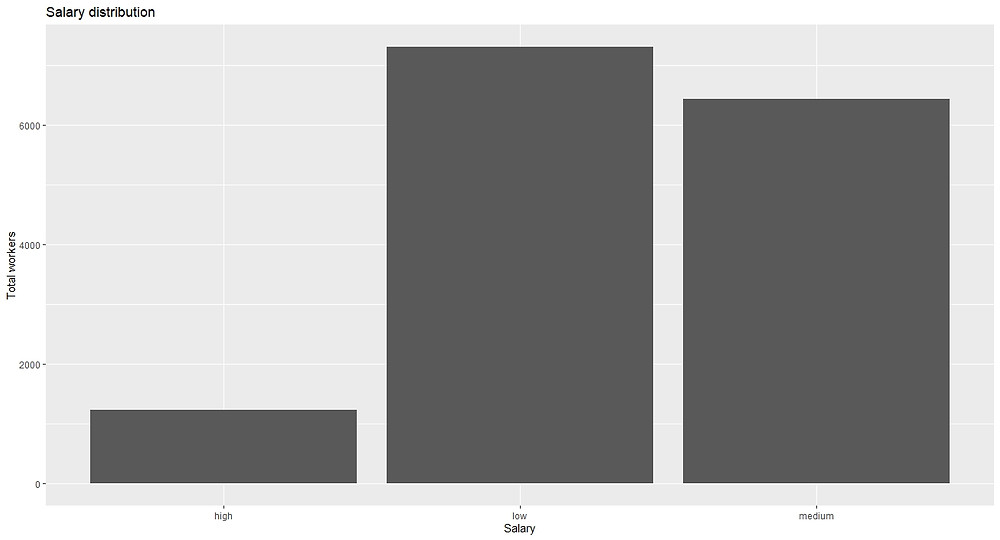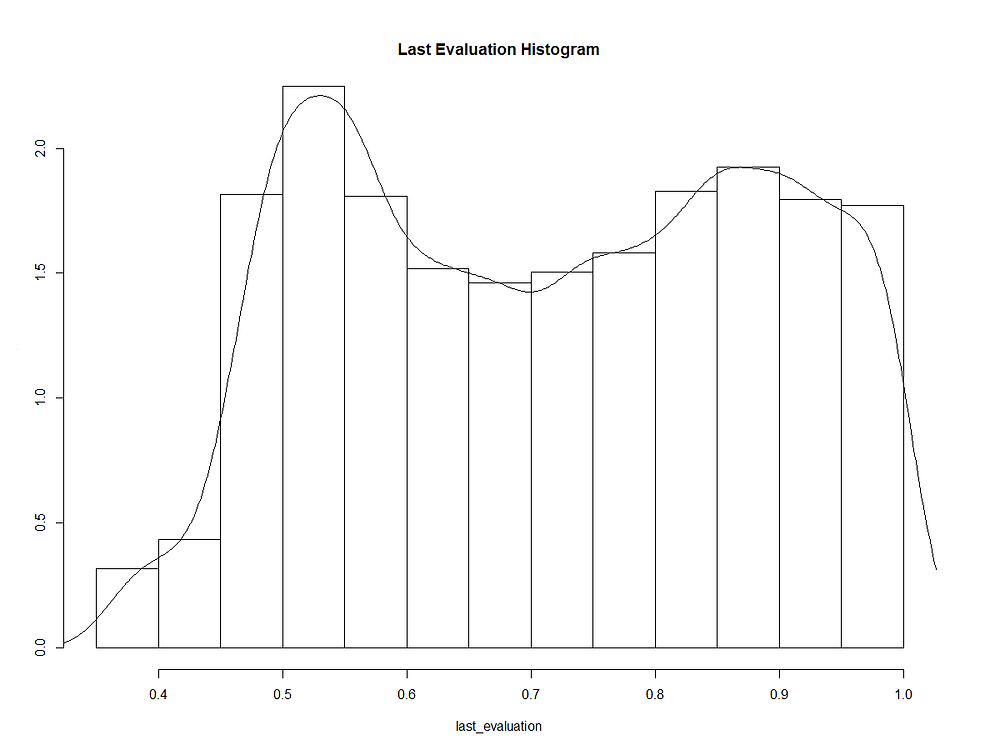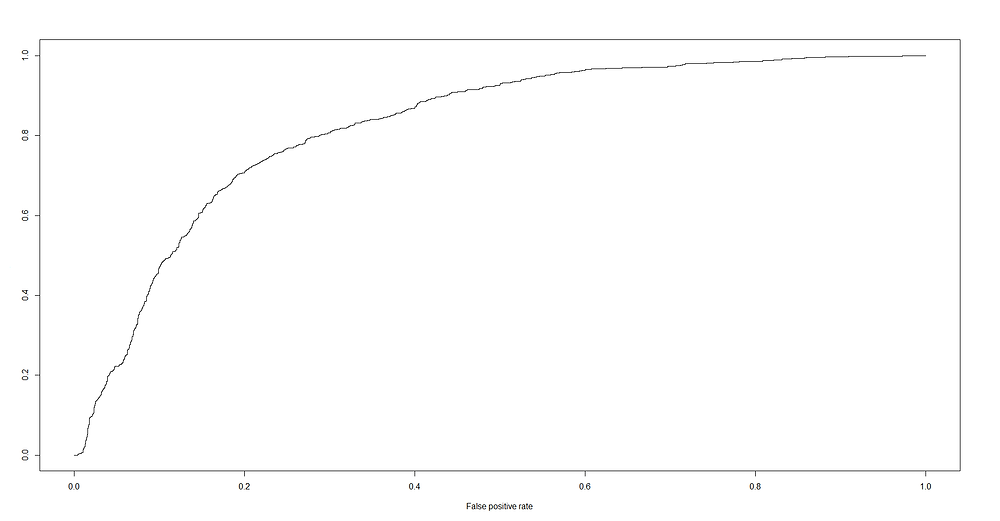Search

Updated: Jul 29, 2020People Analytics

People Analytics is the recognition that employees are the most valuable resource of a company and that, therefore, it is necessary to measure to understand what makes them engaged, productive and happy in the work environment.

There is some resistance on the part of the human resources sector to apply new technologies, according to an American survey, only 4% of companies practice People analytics. We have more and more data that are not being used scientifically to improve the human resources sector.

With People Analytics, we can reduce turnover costs and increase employee engagement and productivity. The analyzes help to correct distortions, to identify high performance professionals with salaries below the market and average performance professionals with salaries above the market.

Objective

The objective of this article is to present a type of applicability of data science in human resources, investigating the causes that cause employees to leave their jobs, seeking to predict which employees may evade the company, so that it is possible to develop some retention policies.

Technology

We will apply the programming language R to perform descriptive analyzes and make predictions with machine learning.

Lifecycle

• Preparing the data

• Exploratory Analysis

• Training Data and Test Data

Below we will be presenting the steps of the data science process for modeling.

Below is the database, where salary information has been categorized to respect privacy policies, without interfering with the quality of the predictive model that meets the business problem proposal that is the subject of this article.Other variables that are also important could be considered in the research, for example, the distance from home to the workplace. However, this sample of this article is able to clearly present the entire process of people analytics.

In other scenarios, we could perform other transformations in the dataset, so that it was possible to create new variables and generate insights through them.

Below we will start the process steps with the entire data science step by step, where our target variable will be "left_company".

Dictionary of variables:

• satisfaction_level: level of employee satisfaction carried out through internal research.

• last_evaluation: last evaluation.

• projects_number: number of projects in which the professional is involved.

• average_hour_per_month: average hours worked in the month.

• time_company: company time.

• work_accident: if you had work accidents.

• left_company: left the company, this being the target variable.

• last_promotion_5years: if the employee has had promotion in the last 5 years.

• area: area in which it operates within the company.

• salary: annual income.

```> # Frequency of the target variable (left_company)
> cbind( Freq = table(left_company),
+  Cumul = cumsum(table(left_company)),
+  relative = round((prop.table(table(left_company))*100),2))
Freq Cumul relative
0 11428 11428 76.19
1 3571 14999 23.81```

Exploratory Analysis```> # Frequency of accidents at work
> cbind( Freq = table(work_accident),
+  Cumul = cumsum(table(work_accident)),
+  relative = round((prop.table(table(work_accident))*100),2))
Freq Cumul relative
0 12830 12830 85.54
1 2169 14999 14.46

> # Last_promotion_5years frequency
> cbind( Freq = table(last_promotion_5years),
+  Cumul = cumsum(table(last_promotion_5years)),
+  relative = round((prop.table(table(last_promotion_5years))*100),2))
Freq Cumul relative
0 14680 14680 97.87
1 319 14999 2.13

> # Employees by department
> vec_dept <- as.vector(area)
> unique(vec_dept)
 "sales" "accounting" "hr"
 "technical" "support" "management"
 "IT" "product_mng" "marketing"
 "RandD"
> vec_dept <- factor(vec_dept)
> qplot(vec_dept, xlab = "Departament", ylab = "Total Colaboradores") + ggtitle("Distribution by Department ")```

Some possible evidence can already be seen during the descriptive analysis, only 2% of employees have had any promotion in the last 5 years and this information can helps us during the predictive model.```> # Employees by salary
> vec_salary <- as.vector(salary)
> unique(vec_salary)
 "low" "medium" "high"
> vec_salary <- factor(vec_salary)
> qplot(vec_salary, xlab = "Salary", ylab = "Total workers") + ggtitle("Salary distribution")
```With more information from the chart above, we realized that the company, at first, does not pay its employees very well and does not give many promotions.

```> # Analyzing the level of satisfaction
> hist(satisfaction_level, freq = F, main = "Level Satisfaction Histogram")
> lines(density(satisfaction_level))``````> # Analyzing the last evaluation
> hist(last_evaluation, freq = F, main = "Last Evaluation Histogram")
> lines(density(last_evaluation))``````> # Analyzing the number of projects
> hist(projects_number, ylim = c(0,0.8), freq = F, main = " Projects Number Histogram")
> lines(density(projects_number))
> # Analyzing the average number of hours worked per month
> hist(average_hour_per_month, freq = F, main = " Histogram of Average Hours Worked per Month ")
> lines(density(average_hour_per_month))
> # Analyzing the average number of hours worked per month
> hist(average_hour_per_month, freq = F, main = " Histogram of Average Hours Worked per Month ")
> lines(density(average_hour_per_month))
> # Analyzing the average number of hours worked per month
> hist(average_hour_per_month, freq = F, main = " Histogram of Average Hours Worked per Month ")
> lines(density(average_hour_per_month))
> # Analyzing the average number of hours worked per month
> hist(average_hour_per_month, freq = F, main = " Histogram of Average Hours Worked per Month ")
> lines(density(average_hour_per_month))
> # Analyzing the average number of hours worked per month
> hist(average_hour_per_month, freq = F, main = " Histogram of Average Hours Worked per Month ")
> lines(density(average_hour_per_month))

```Some indicators as in the graph above, we need market parameters, such as the number of projects that each employee works at the same time, in order to understand if there is an overload of work Some.

```> # Analyzing the average number of hours worked per month
> hist(average_hour_per_month, freq = F, main = " Histogram of Average Hours Worked per Month ")
> lines(density(average_hour_per_month))
> # Time working in the company
> hist(time_company, ylim = c(0,1.2), freq = F, main = " Histogram of Time Worked in the Company ")
> lines(density(time_company))```The chart above demonstrates something that we should consider, as we have identified a considerable proportion of employees who work long hours and this may be an alert that we will consider later.

```> # Time working in the company
> hist(time_company, ylim = c(0,1.2), freq = F, main = " Histogram of Time Worked in the Company ")
> lines(density(time_company))```In the chart above, we noticed that from the fourth to the fifth year the employees start to leave the company.

Then we will perform a correlation analysis, where 1 the variables have a strong correlation and -1 have a negative correlation and 0 have no correlation.

```> # Calculating the correlation
> par(mar=c(4,3,2,2))
> par(oma=c(1,1,2,2))
> corrplot(cor(hr[,c(1,2,3,4,5,6,7,8)]), type = "lower", tl.col = "black", method = "ellipse")```Satisfaction level has the highest negative correlation with the target variable.

```> # Leaving the company x Accident at work – Barplot
> t <- table(left_company, hr\$ work_accident)
> barplot(prop.table(t,2), legend = paste(unique(left_company)),
+  ylab = "Accumulated Probability", xlab = "Accident at Work")```

In the list below, we correlate work accidents with leaving the company, 0 represents "no work accidents" and 1 represents "work accidents", the dark gray color means that the employee leaves the company. We believe that there is a bivariate correlation because when there is an accident at work, the occurrence of leaving the company is greater.We will then perform the same bivariate analysis for the other variables through the cross table.

```> # Exit from the company x Accident at work – Crosstable
> CrossTable(left_company, hr\$ work_accident, prop.r = TRUE, prop.c = FALSE,
+ prop.t = TRUE, prop.chisq = FALSE)``````> # Exit from the company x Promotion in the last 5 years – Crosstable
> CrossTable(left_company, last_promotion_5years, prop.r = TRUE, prop.c = FALSE,
+  prop.t = TRUE, prop.chisq = FALSE)``````> # Exit from company x Salary – Aggregate
> aggregate(left_company ~ salary, FUN = mean)
salary left_company
1 high      0.06628941
2 low       0.29688354
3 medium    0.20431275```

we noted above in the table above that the highest percentage of leaving the company is more concentrated in the low wages group.

```> # Exit from the company x Area – Aggregate
> aggregate(left_company ~ area, FUN = mean)
area left_company
1   accounting    0.2659713
2           hr    0.2909337
3           IT    0.2224939
4   management    0.1444444
5    marketing    0.2365967
6  product_mng    0.2195122
7        RandD    0.1537484
8        sales    0.2449275
9      support    0.2489906
10   technical    0.2562500```

We observed in the table above that the human resources sector is the one with the highest average turnover

Initial Conclusion

Therefore, initially we identified possible causes of turn over in the company, they are:

• People who haven't had a promotion in the past 5 years have left more than those who have.

• People in the Management area have the lowest average exit from the company and HR people the highest average.

• People with low salaries have a higher average exit from the company compared to other categories.

Creating the predictive model

Training Data and Test Data

• Logistic Regression Model

```> # Dividing 70% for training and 30% for testing
> set.seed(4)
> hr_train <- sample(nrow(hr), floor(nrow(hr)*0.7))
> train <- hr[hr_train,]
> test <- hr[-hr_train,]

##### -------------- Logistic Regression ---------------

# Testing all variables
names(hr)
model <- glm(formula = (left_company) ~  satisfaction_level
+ last_evaluation
+ projects_number
+ average_hour_per_month
+ time_company
+ work_accident
+ last_promotion_5years
+ area
+ salary,
family = binomial(logit), data = train)```

All variables are relevant, the most important being the level of satisfaction, work accident and salary.

```> # Forecasts
> p <- predict(model, test, type = "response")
> pr <- prediction(p, test\$left_company)
> # Calculating the rate of true positive and false positive
> prf <- performance(pr, measure = "tpr", x.measure = "fpr")
> plot(prf)```In the graph above, the closer the curve is to 1 on the y-axis, the more accurate and predictive.

Then we calculate the area of the graph to quantify the accuracy of the predictive model below.

```> # Area Under the Curve
> auc <- performance(pr, measure = "auc")
> auc <- auc@y.values[]
> auc
 0.8224268```

• Decision Tree Model

```> ##### -------------- Decision tree ---------------
>
> # Using the same combination of variables
> tree1 <- rpart(formula = (left_company) ~ satisfaction_level
+ last_evaluation
+ projects_number
+ average_hour_per_month
+ time_company
+ work_accident
+ last_promotion_5years
+ area
+ salary,
data = train,
method = "class")```

```> # Plot
> plot(tree1, uniform = TRUE, main = "Classification Tree")
> text(tree1, use.n = TRUE, all = TRUE, cex = .8)
> printcp(tree1)```After assembling the decision tree above, we analyze the errors below to identify whether there is a need to prune the tree

```> tree1\$cptable[which.min(tree1\$cptable[,"xerror"]),"CP"]
 0.01
> plotcp(tree1)```It will not be necessary to prune the tree

Then we perform the same tests performed for logistic regression and apply a decision tree model.```> # Confusion Matrix - Training Data
> conf_matrix_tree <- table(train\$left_company, predict(tree1, type = "class"))
> rownames(conf_matrix_tree) <- paste("Actual", rownames(conf_matrix_tree), sep = ":")
> colnames(conf_matrix_tree) <- paste("Pred", colnames(conf_matrix_tree), sep = ":")
> print(conf_matrix_tree)

Pred:0 Pred:1
Actual:0     7928    90
Actual:1     220     2261```

```> # Test Data
> test_tree = predict(tree1, test, type = "prob")
> # Storing the model's performance score
> pred_tree <-prediction(test_tree[,2], test\$left_company)
> # Area under the Curve
> perf_tree <- performance(pred_tree,"auc")
> perf_tree
A performance instance
'Area under the ROC curve'

# Calculating the rate of true positive and false positive
> perf_tree <- performance(pred_tree, "tpr", "fpr")
> # Plot Curva ROC
> plot(perf_tree, lwd = 1.5)```

Conclusion

1- What are the most important aspects that are decisive for employees to leave their jobs?

In the logistic regression, I found satisfaction level, work accident and salary as the most relevant aspects.

The decision tree, the most important ones are the level of satisfaction, the time spent in the company and the number of projects.

2- What is the best way to make these predictions: logistic regression or decision tree?

For this data set, the decision tree performed better on the test data set.

We can see this from the ROC curves below.26 views